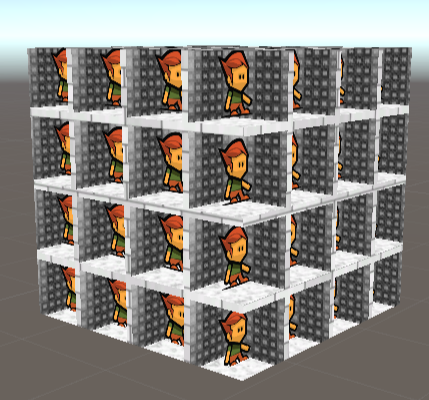# 简介

Interior Mapping主要用于在物体表面利用透视错局模拟出格子结构的内部内容。虽然从外部观察起来，物体内部的构造是“真实的”，但一切渲染都没有依靠更多的模型或真实顶点。这在渲染大量模式化的内部结构中会有很大作用。

1.房间的数量不会影响framerate或内存开销
2.并不需要太多的额外资源就可以表现大的场景

# 基本思路的实现2.还没有加上sprite层（家具层）丰富室内内容
3.在拐角处会看到本不应该看到的墙面

Block: 每个单独的房间称为一个Block

d: 代表一个Block某个方向上的长度
_BoundarySize: 指一个Cube Mesh的某条棱的长度
_Tiling: 指在某个坐标轴方向上，Mesh被切分的次数# 可配置厚度墙面的实现## 判断像素是否在墙内## 使用AND还是OR# 制作Sprite Layer

## 朴素的截面偏移层### 支持多方向适配## 类Billboard Sprite Layer

### 基础知识准备

$$\left[ \begin{array}{cccc} x_{clip}\\ y_{clip}\\ z_{clip}\\ 1 \end{array} \right ] = \left[ \begin{array}{cccc} \frac{ {2n} }{ {r-l} } & 0 & \frac{ {r+l} }{ {r-l} } & 0 \\ 0 & \frac{ {2n} }{ {t-b} } & \frac{ {t+b} }{ {t-b} } & 0 \\ 0 & 0 & \frac{ {-(f+n)} }{ {f-n} } & \frac{ {-2fn} }{ {f-n} } \\ 0 & 0 & -1 & 0 \\ \end{array} \right ] \left[ \begin{array}{cccc} x_{view}\\ y_{view}\\ z_{view}\\ 1 \end{array} \right ]$$NDC还有另外一个特点：该空间中如果要计算任意一点的属性值，直接根据Barycentric Interpolation来插值的话会因为深度的非均匀压缩而产生扭曲$$p=z[\frac{p_1}{z_1}(1-t) + \frac{p_2}{z_2}t]$$

Perspective Divide的矩阵表示
$$\left[ \begin{array}{cccc} x_{ndc}\\ y_{ndc}\\ z_{ndc} \end{array} \right ] = \left[ \begin{array}{cccc} \frac{1}{-z_{view}}\\ \frac{1}{-z_{view}}\\ \frac{1}{-z_{view}} \end{array} \right ]^{\mathsf{T}} \left[ \begin{array}{cccc} x_{clip}\\ y_{clip}\\ z_{clip} \end{array} \right ]$$$$[M_{Proj}] = \left[ \begin{array}{cccc} \frac{1}{-z_{view}}\\ \frac{1}{-z_{view}}\\ \frac{1}{-z_{view}}\\ N/A \end{array} \right ]^{\mathsf{T}} \left[ \begin{array}{cccc} \frac{ {2n} }{ {r-l} } & 0 & \frac{ {r+l} }{ {r-l} } & 0 \\ 0 & \frac{ {2n} }{ {t-b} } & \frac{ {t+b} }{ {t-b} } & 0 \\ 0 & 0 & \frac{ {-(f+n)} }{ {f-n} } & \frac{ {-2fn} }{ {f-n} } \\ 0 & 0 & -1 & 0 \end{array} \right ]$$### 让Billboard跟随物体### 让Billboard带透视### Tiled Sprite Layer# 正确显示边角的遮挡# 后面的改进

1.通过事先bake好光照，使室内的表现更加真实。计算真实的光照也是有可能的，只不过可能需要更多的操作，需要权衡一下是否真的需要。
2.处理好不同方向的偏移补偿，目前的uv offset补偿其实是有问题的，没有考虑顶部和底部的情况。（不过如果大楼是封顶的倒也不太要紧）
3.本文使用的是分开的墙面texture方法，也有些实现使用的是Cubemap。